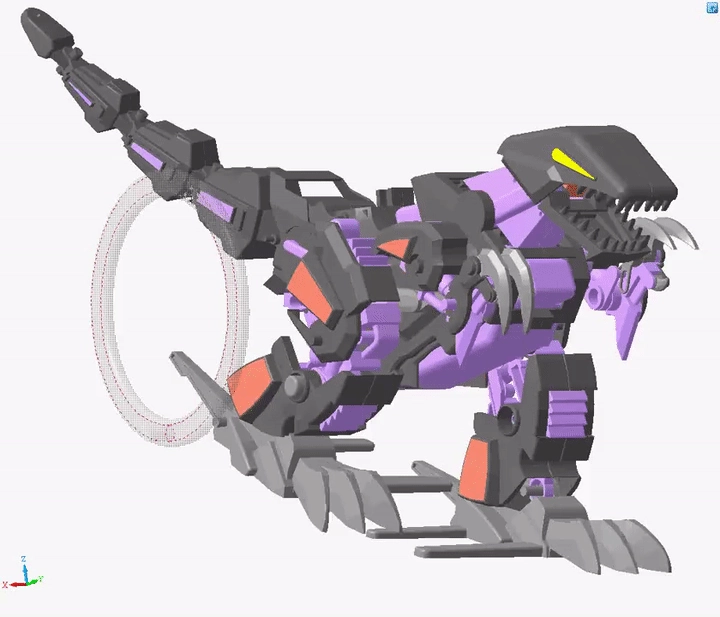# C3D Solver

Define dimensions and constraints to create connections between geometric elements.

Constraints represent all relationships among geometric objects in 2D/3D applications. These include dimensions that define angles and distances and logical constraints that define concepts like a coincidence, parallelism, perpendicularity, tangency, etc.

Using C3D Solver, developers incorporate dimensions and logical constraints in their applications to create connections between geometric objects in 2D and 3D models. C3D Solver maintains constraints as users make changes to the geometry. For example, when users modify dimensions, C3D Solver instantly recalculates all depending geometric objects and maintains existing relationships.

Our C3D Solver reduces 2D/3D modeler development costs significantly, as the constraint engine is ready-made for you. You can plug the C3D Solver in at any stage of your product development cycle.

## High Performance

C3D Solver was written for performance, and we are always working at finding new ways to further speed up the code by simplifying the math, dividing analysis into sub-tasks, using large sparse matrices, and so. One key productivity boost, for instance, is the Planner, which extracts from the entire set of constraints a smaller subset sufficient to do the job.## Live Constraint Verification

Splitting the initial model of constraints into sub-tasks not only improves the speed at which the solution arrives but also identifies a wide variety of situations in which the built-in diagnostics avoid contradictions in the system of constraints. This assists users in constructing high-quality parametric models without avoiding contradictions in the system of constraints.Porting the 2D solver to JavaScript unveils new application possibilities for C3D Solver, such as the development of cloud solutions for doing online sketches, interior planning with driving dimensions, and so on. This makes it possible to quickly implement a 2D drawing editor in a browser complete with dimensioning and constraints. Perhaps most significantly, the 2D solver for JavaScript is a platform-independent solution.

## C3D Solver Capabilities

C3D Solver Applications:

• creating 2D parametric sketches with driving dimensions and constraints;
• positioning bodies in assemblies using constraints and dimensions in 3D;
• rebuilding changed models while keeping previously defined constraints intact;
• modeling planar and spatial mechanisms;
• creating 3D wireframes.

Dimensional Constraints in C3D Solver:

• dimensions by type of calculation: driving, interval and variable dimensions;
• dimensions in length units: distance, radial and diameter, curve length;
• angular dimensions.

2D and 3D Functions:

• creating and solving parametric constraints;
• manipulating geometry;
• dragging geometry;
• satisfying constraints;
• analyzing degrees of freedom (2D only);
• clustering rigid sets (3D only);
• journaling API calls.

Logical Constraints in C3D Solver

General:

• coincidence;
• fixed geometry;
• parallelism;
• perpendicularity;
• reflection symmetry;
• tangency.

2D Constraints:

• alignment;
• point on a curve;
• equal length;
• fixed length and direction;
• fixed spline derivatives;
• horizontal position;
• vertical position;
• bisector;
• smooth G1 and G2 transitions;
• offset curves.

3D Constraints:

• coaxiality;
• user-defined dependencies;
• linear and angular patterns;
• mechanical transmissions;
• cam mechanisms;
• rack and gear mechanisms.